### My Introduction to Scheme – part 2

In my last post, I started to look at the Scheme language. We finished up with a simple if statement. Its syntax is like this: (if (condition) result1 result2).

In our last example, “result1” and “result2” were simple return types. If you wanted, the “result1” or “result2” could also be functions that are evaluated to get a return value. Let’s look at that.

In this case, if 4 is greater than 0, we are going to multiply 4 times 4, if 4 isn’t greater than 0, we are just going to add 4 plus 4.

`(if (> 4 0) (* 4 4) (+ 4 4))`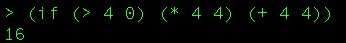You can see that because 4 is greater than 0, it executed the first block which evaluated 4 * 4 which is 16. Like last time, here is the equivalent C# version to hopefully add clarity.

```if (4 > 0)
{
return 4 * 4;
}
else
{
return 4 + 4;
}
```

One of the most powerful things you can do in a functional language is – of course – to define your own functions. Here is a simple function that defines a function named pete that squares a number passed to it.

`(define pete (lambda (x) (* x x)))`

So here, we are calling the define language construct (which is how you declare variables, functions, etc in Scheme) and defining “pete” which is a lambda that takes one parameter – x – and returns the output of the * function being passed x and x. You see that if we call the pete function and pass in 7, it returns 49 as you would expect.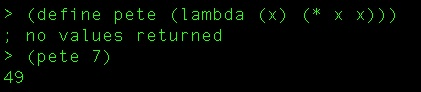Functions are first class citizens in Scheme, so you can pass them around as arguments. Let’s see this example where I’m going to let us “do-stuff-to-four-and-nine”. My goal here is that we are going to create a function that takes a function as a parameter. It then calls that function, passing it 4 and 9.

So, first the function. (I used a hard return in the REPL window so that the screenshot below would definitely fit. It does not cause a problem in the language to have it there).

`(define do-stuff-to-four-and-nine (lambda (our-func) (our-func 4 9)))`All I’ve done is define “do-stuff-to-four-and-nine” as a function that takes a parameter (our-func) that I then use in the body of the lambda, calling it and passing 4 and 9 as arguments. Next, let’s define 4 functions that we can pass to our “do stuff” function.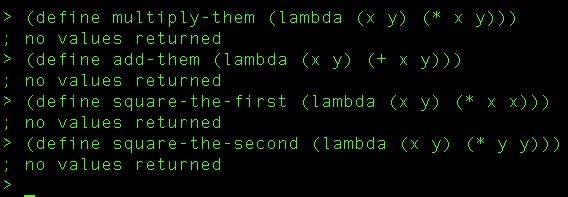You should be able to simply read these by now. All four take two parameters, and are plainly named for the simple operations they perform on them.

Now, all that is left is to call the “do-stuff” function and pass it each of these functions in turn.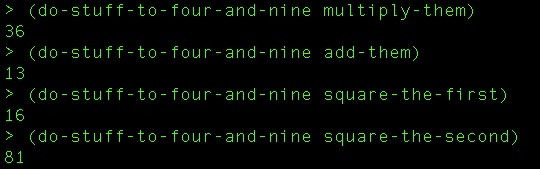Interestingly, the function must take the right number of arguments. If not, the language throws up on you. Notice here that I define a function that only takes one argument. Then when I try to pass it in to our “four and nine” function, I get an error.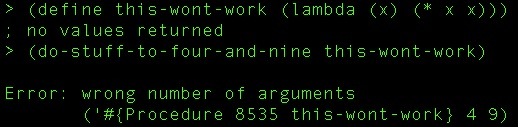I hope that you’ve learned a little bit more about how Scheme works and how powerful it can be. My next post is going to take a look at recursion in Scheme and putting a lot of these lessons together.